stat_prop() is a variation of ggplot2::stat_count() allowing to compute custom proportions according to the by aesthetic defining the denominator (i.e. all proportions for a same value of by will sum to 1). The by aesthetic should be a factor. If by is not specified, proportions of the total will be computed.

stat_prop(
mapping = NULL,
data = NULL,
geom = "bar",
position = "fill",
...,
width = NULL,
na.rm = FALSE,
orientation = NA,
show.legend = NA,
inherit.aes = TRUE,
complete = NULL
)

## Arguments

mapping

Set of aesthetic mappings created by aes(). If specified and inherit.aes = TRUE (the default), it is combined with the default mapping at the top level of the plot. You must supply mapping if there is no plot mapping.

data

The data to be displayed in this layer. There are three options:

If NULL, the default, the data is inherited from the plot data as specified in the call to ggplot().

A data.frame, or other object, will override the plot data. All objects will be fortified to produce a data frame. See fortify() for which variables will be created.

A function will be called with a single argument, the plot data. The return value must be a data.frame, and will be used as the layer data. A function can be created from a formula (e.g. ~ head(.x, 10)).

geom

Override the default connection with ggplot2::geom_bar().

position

Position adjustment, either as a string naming the adjustment (e.g. "jitter" to use position_jitter), or the result of a call to a position adjustment function. Use the latter if you need to change the settings of the adjustment.

...

Other arguments passed on to layer(). These are often aesthetics, used to set an aesthetic to a fixed value, like colour = "red" or size = 3. They may also be parameters to the paired geom/stat.

width

Bar width. By default, set to 90% of the resolution() of the data.

na.rm

If FALSE, the default, missing values are removed with a warning. If TRUE, missing values are silently removed.

orientation

The orientation of the layer. The default (NA) automatically determines the orientation from the aesthetic mapping. In the rare event that this fails it can be given explicitly by setting orientation to either "x" or "y". See the Orientation section for more detail.

show.legend

logical. Should this layer be included in the legends? NA, the default, includes if any aesthetics are mapped. FALSE never includes, and TRUE always includes. It can also be a named logical vector to finely select the aesthetics to display.

inherit.aes

If FALSE, overrides the default aesthetics, rather than combining with them. This is most useful for helper functions that define both data and aesthetics and shouldn't inherit behaviour from the default plot specification, e.g. borders().

complete

Name (character) of an aesthetic for those statistics should be completed for unobserved values (see example)

## Value

A ggplot2 plot with the added statistic.

## Aesthetics

stat_prop() understands the following aesthetics (required aesthetics are in bold):

• x or y

• by (this aesthetic should be a factor)

• group

• weight

## Computed variables

count

number of points in bin

prop

computed proportion

vignette("stat_prop"), ggplot2::stat_count()

## Examples

library(ggplot2)
d <- as.data.frame(Titanic)

p <- ggplot(d) +
aes(x = Class, fill = Survived, weight = Freq, by = Class) +
geom_bar(position = "fill") +
geom_text(stat = "prop", position = position_fill(.5))
p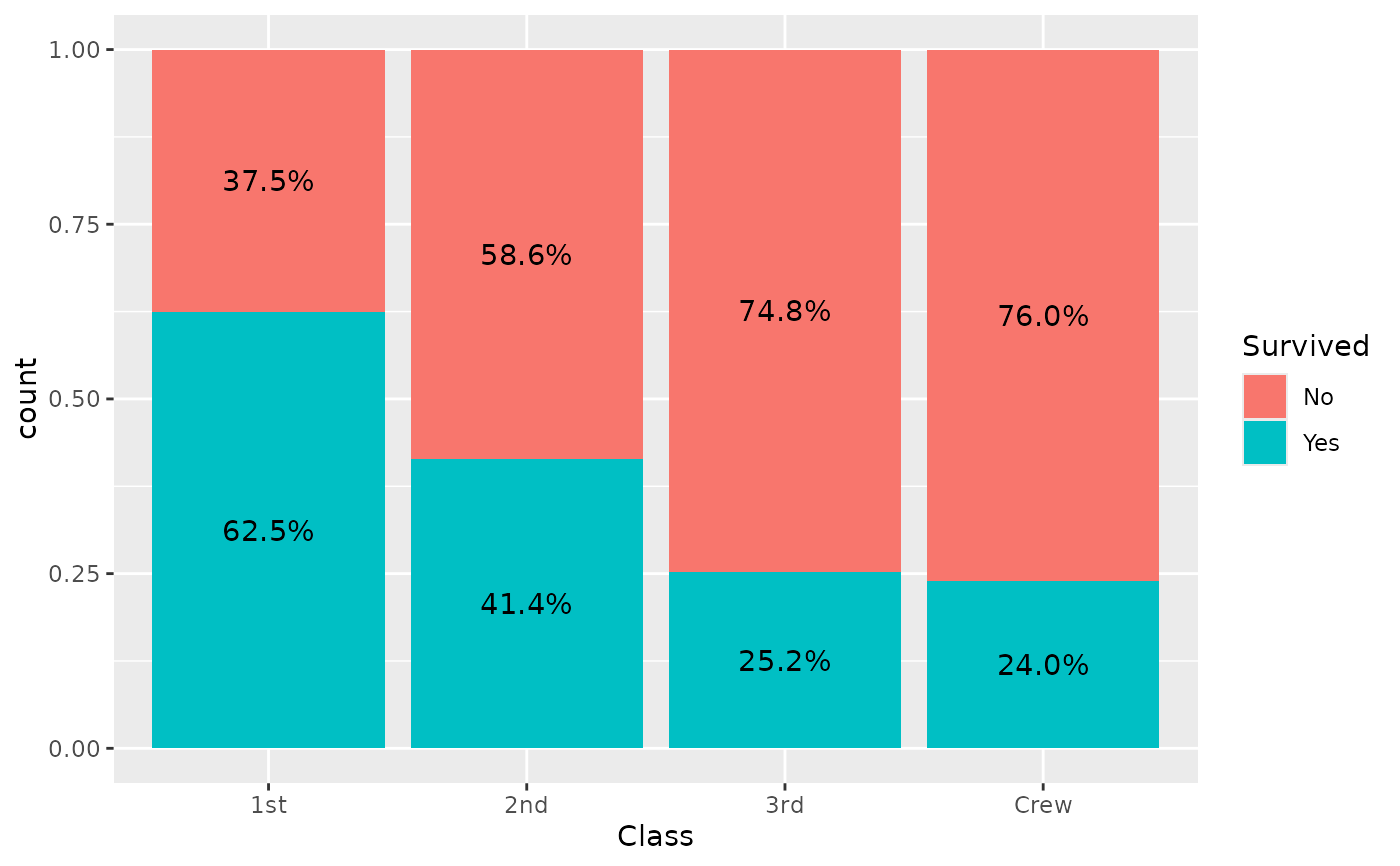p + facet_grid(~Sex)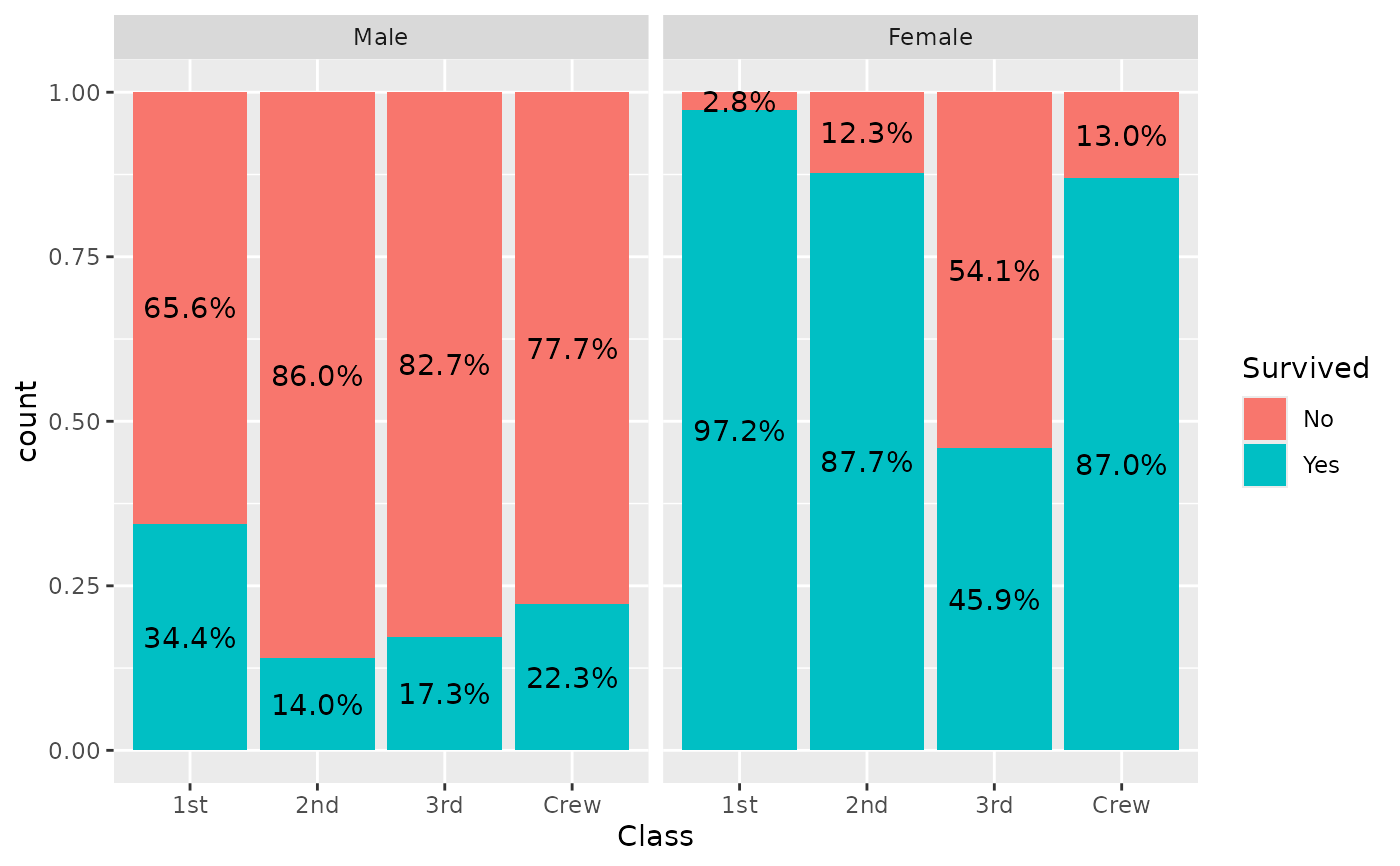ggplot(d) +
aes(x = Class, fill = Survived, weight = Freq) +
geom_bar(position = "dodge") +
geom_text(
aes(by = Survived),
stat = "prop",
position = position_dodge(0.9), vjust = "bottom"
)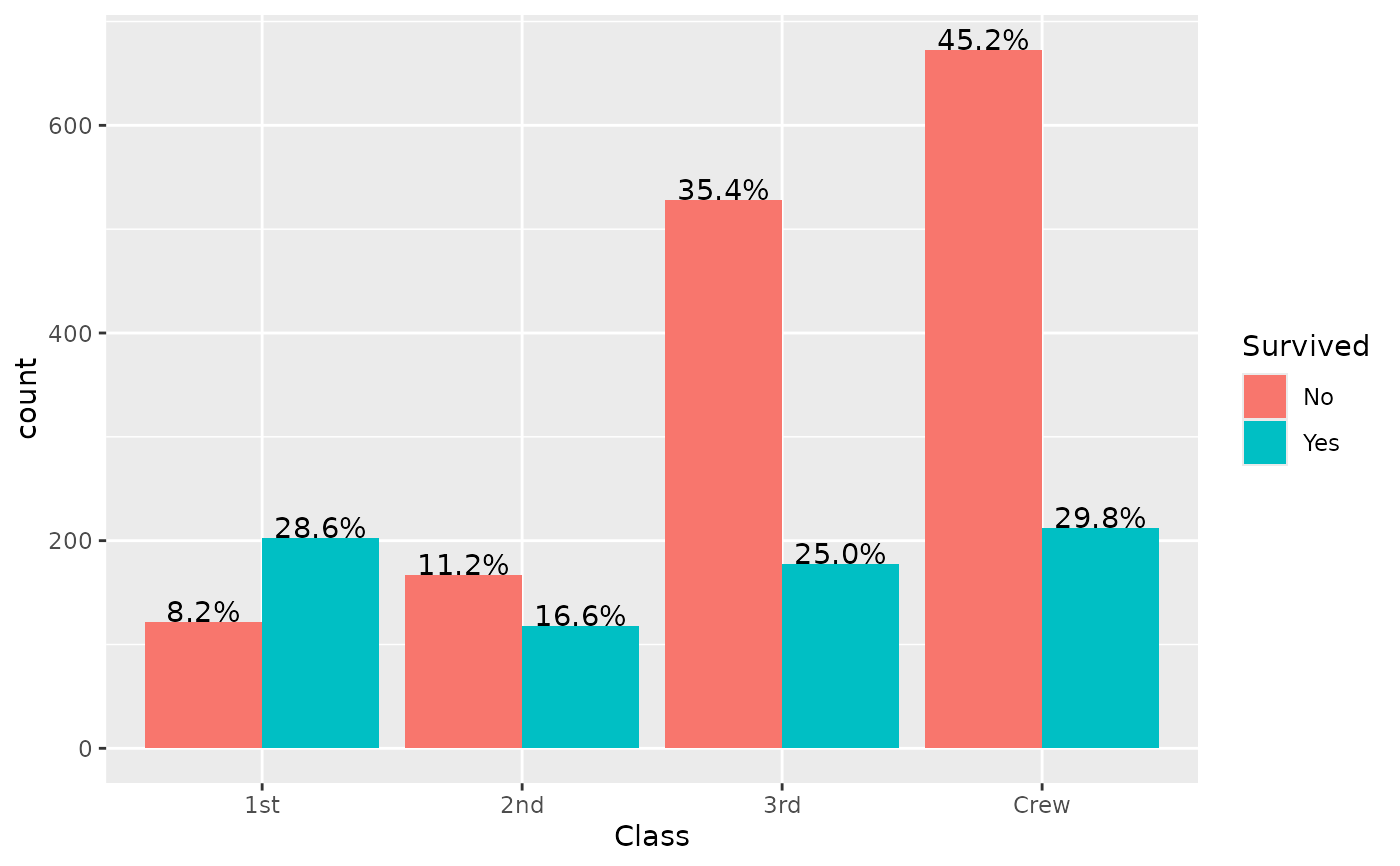# \donttest{
if (requireNamespace("scales")) {
ggplot(d) +
aes(x = Class, fill = Survived, weight = Freq, by = 1) +
geom_bar() +
geom_text(
aes(label = scales::percent(after_stat(prop), accuracy = 1)),
stat = "prop",
position = position_stack(.5)
)
}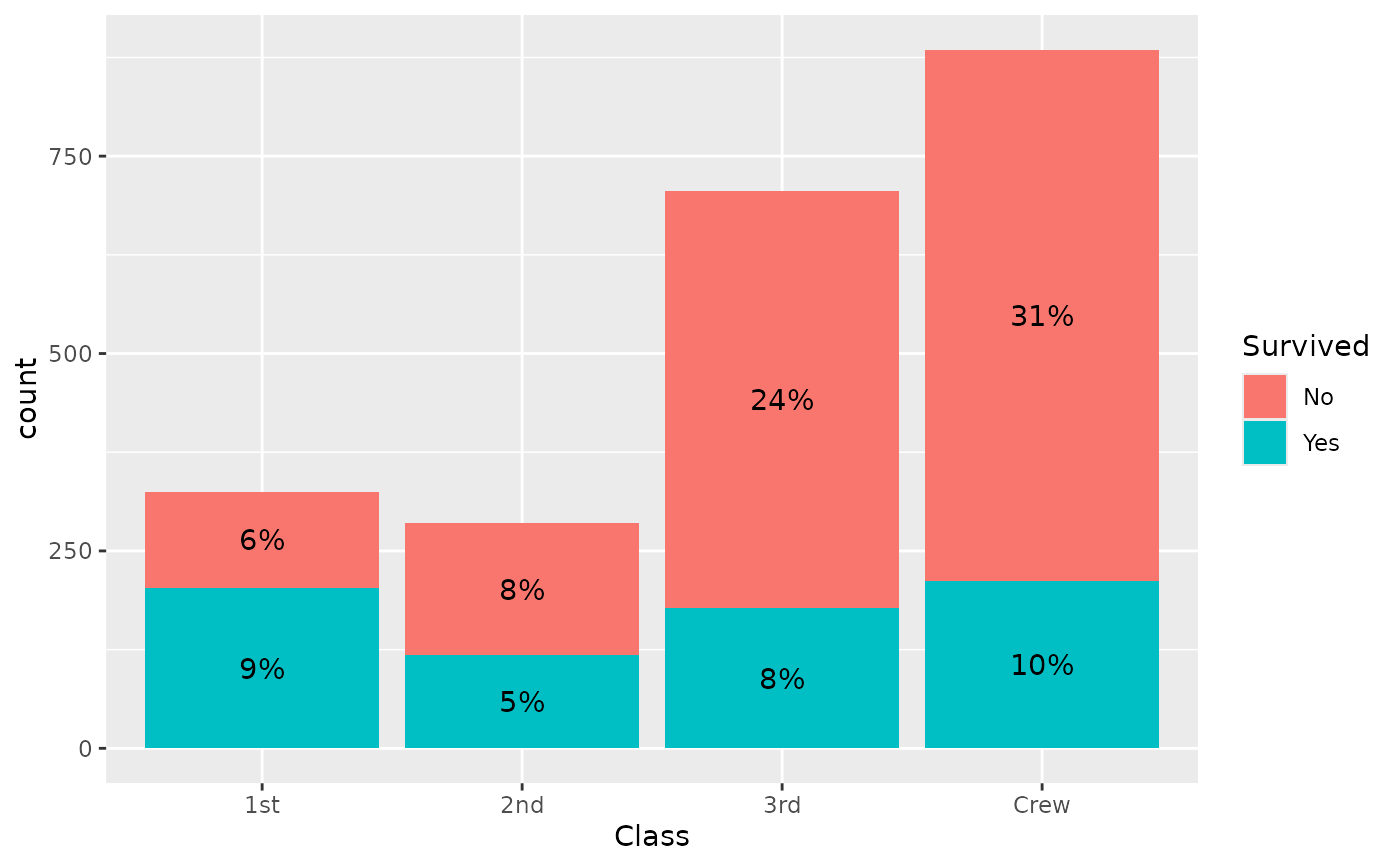# displaying unobserved levels with complete
d <- diamonds %>%
dplyr::filter(!(cut == "Ideal" & clarity == "I1")) %>%
dplyr::filter(!(cut == "Very Good" & clarity == "VS2")) %>%
dplyr::filter(!(cut == "Premium" & clarity == "IF"))
p <- ggplot(d) +
aes(x = clarity, fill = cut, by = clarity) +
geom_bar(position = "fill")
p + geom_text(stat = "prop", position = position_fill(.5))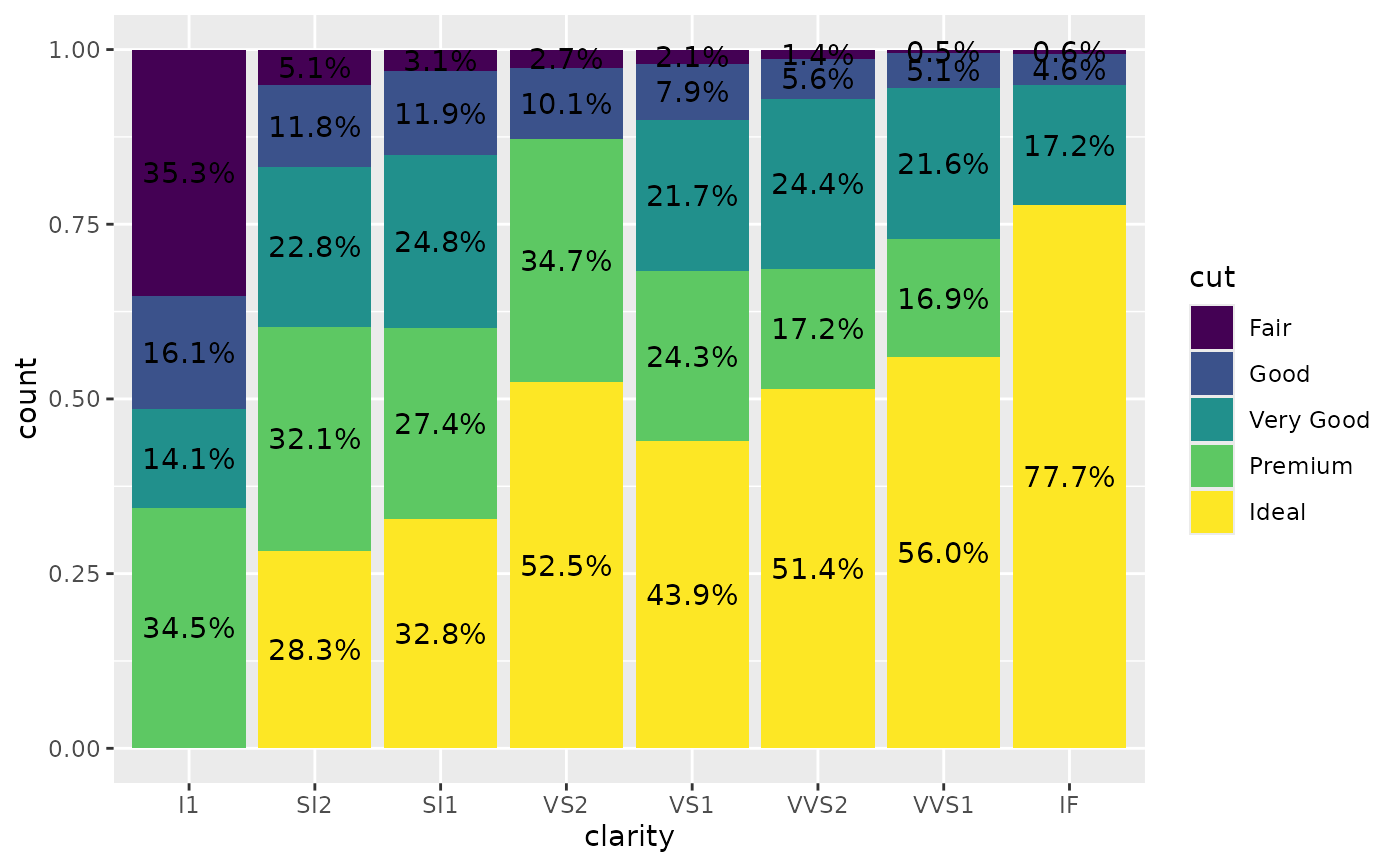p + geom_text(stat = "prop", position = position_fill(.5), complete = "fill")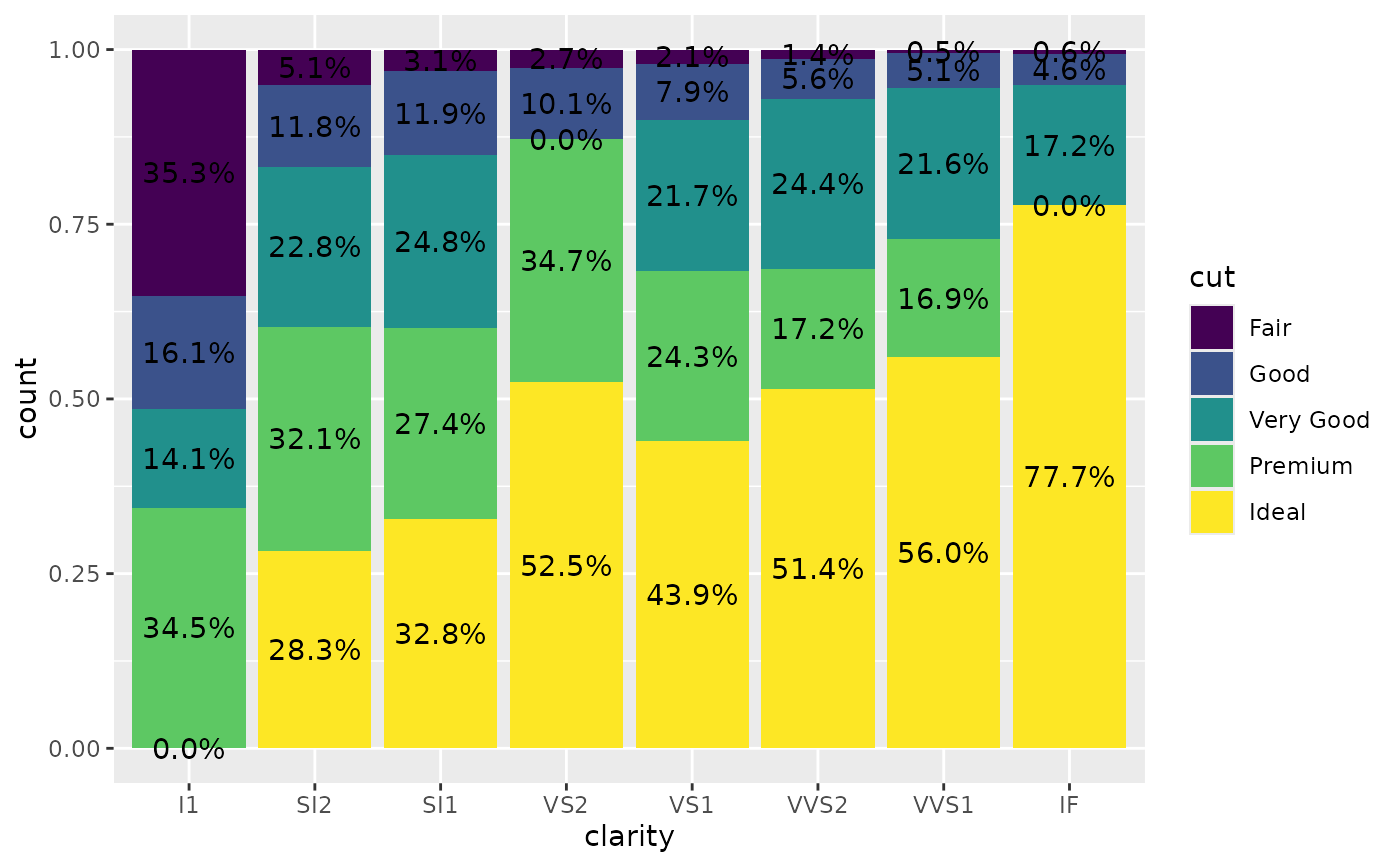# }